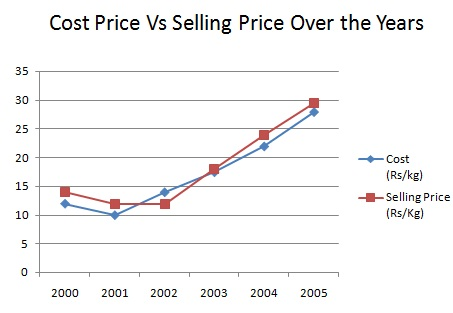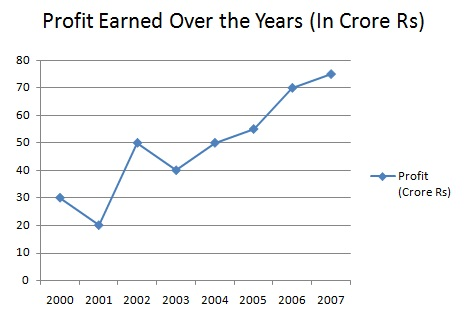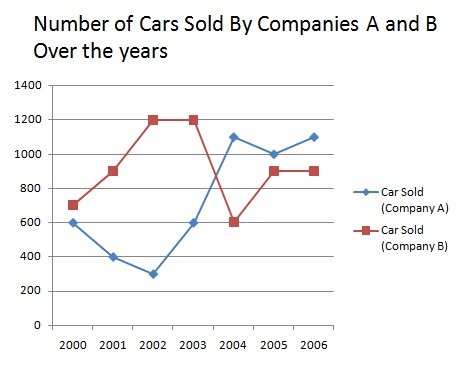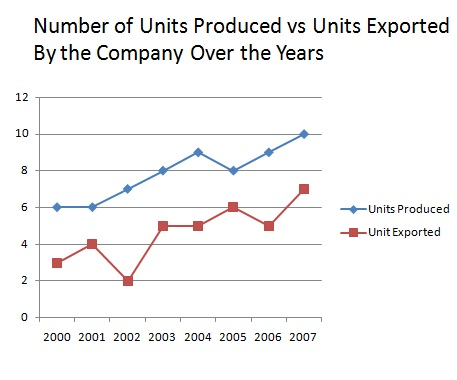# Line Charts - Online Quiz

Following quiz provides Multiple Choice Questions (MCQs) related to Line Charts. You will have to read all the given answers and click over the correct answer. If you are not sure about the answer then you can check the answer using Show Answer button. You can use Next Quiz button to check new set of questions in the quiz.Directions: The chart given underneath demonstrates the assembling volume (in kg), expense value (Rs. /kg) and offering value (Rs. /kg) of an article over year. Study the chart and answer the question given below:Q 1 - In which of the next years was the expense/deal value proportion the most minimal?

A - 2003

B - 2000

C - 2005

D - None of these

Explanation

```expense and deals value proportion :
2000= 12/14 =0.857,
2001 = 10/12 = 0.833,
2002 = 14/12 = 7/6,
2003 = 18/17.5 = 1.028
2004 =22/24 = 0.916,
2005 = 28/29.5 = 0.949
```

Directions: The chart given underneath demonstrates the assembling volume (in kg), expense value (Rs. /kg) and offering value (Rs. /kg) of an article over year. Study the chart and answer the question given below:Q 2 - What was the expand/diminish in benefit from 2001 to 2004?

A - Rs. 300 build

B - Rs. 320 build

C - Rs. 30 diminish

D - Rs. 20 increment

Explanation

```benefit in 2001 = (12-10*150) = Rs. 300
Benefit in 2004 = Rs [(24-22)*160]= Rs. 320
Increment in benefit = Rs. (320-300) = Rs. 20
```

Directions: Study the graph below carefully and answer the following:Q 3 - In which year the % expansion in the benefit from that of the earlier year is the most elevated?

A - 2000

B - 2002

C - 2004

D - 2006

Explanation

```gain % in:
2002 = {(50-20/20)*100} % = 150%,
2004 = {(50-40/40)*100} %= 25%,
2005 = {(55-50/50)*100} % = 10%,
2006 = {(70-55/55)*100} % = 27.3%,
2007 = {(75-70/70)*100} % = 7.1%
So, the gain% is highest in 2002.
```

Directions: Study the graph below carefully and answer the following:Q 4 - What is the inexact % expansion in the benefit of the year 2004. On the off chance that the pay of that year was Rs. 120 crore?

A - 12%

B - 4%

C - 7%

D - 15%

Explanation

```Increase% in gain in 2007
= {(75-70)/70*100} %
= (5/70*100) %
= 7%
```

Directions: Study the graph below carefully and answer the following:Q 5 - Deals in the year 2004 for organization A structures what % of aggregate offers of organization A for every one of the years together?

A - 19.64%

B - 18.30%

C - 22.46%

D - 24.16%

Explanation

```Total sale of Company A in 2004  =1100
Total sale of A during These years = (600+900+300+600+1100+1000+1100)= 5600
Required % = (1100/5600*100) % = 19.64%
```

Directions: Study the graph below carefully and answer the following:Q 6 - Which of the accompanying mixes of year and% ascend in deals from the earlier year for organization is right?

A - 2003= 99.85

B - 2004 = 83.33

C - 2001 = 43.21

D - 2006 = 7.68

Explanation

```percent rise in sales in 2003 = {(600-300/600)*100}% = 100%
Percent rise in sales in 2004 = {(1100-600/600)*100} % = 83.33%
∴ Rise in 2004 by 83.33% is correct.
```

Directions: Study the graph below carefully and answer the following:Q 7 - What is the proportion of aggregate offers of organization A to the aggregate offers of organization B individually throughout the years?

A - 26:29

B - 27:31

C - 53:51

D - none of these

Explanation

```Required number = 5600:5900 =  56:59
```

Directions: Study the graph below carefully and answer the following:Q 8 - What is the normal number of units sent out throughout the years?

A - 27857000

B - 462500000

C - 46250000

D - 278570000

Explanation

```Average number of units exported over the year
= 1/8 (3+4+2+5+5+6+5+7) = 37/8 crores
= 37/8*10000000 = 37* 1250000 = 46250000.
```

Directions: Study the graph below carefully and answer the following:Q 9 - In which year the percent of units sent out to the units delivered is the most extreme?

A - 2007

B - 2005

C - 2003

D - 2001

Explanation

```Percentage of units exported to units produced in various years is given by:
2000 = (3/6*100) % = 50%, 2001 = (4/6*100) %= 66.6%
2002= (2/7*100) % = 28.5%, 2003 = (5/8*100) % = 62.5%
2004 = (5/9*100) % = 55.5%, 2005 = (6/8*100) % = 75%
2006 = (5/9*100) % = 55.5%, 2007 = (7/10*100) % = 70%
So, it is the maximum in the year 2005
```

Directions: Study the graph below carefully and answer the following:Q 10 - In which year, the percent ascend in units delivered from the former year, is the most extreme?

A - 2007

B - 2006

C - 2003

D - 2002

Explanation

```Required %  in
2002 = {(7-6)/6* 100} % = 16.67%,
2003 = {(8-7)/7*100} % =14.28%,
2004 = {(9-8)/8* 100} % =12.5%,
2006 ={(9-8)/8* 100}% =12.5%
2007 = {(10-9)/9* 100} % =11.1%
∴ It is maximum in the year 2002.
```
aptitude_line_charts.htm